Feature Articles: Basic Research Envisioning Future Communication

# Learning from a Large Number of Feature Combinations

## Abstract

Second-order polynomial regression can often outperform simple linear regression by making use of feature combinations. However, when the number of feature combinations is large, second-order polynomial regression quickly becomes impractical. In this article, we present convex factorization machines, a new technology developed by NTT Communication Science Laboratories, which can cope with a large number of feature combinations and guarantees globally optimal model parameters.

Keywords: machine learning, regression analysis, feature combinations

## 1. Introduction

With the democratization of the Internet, social media, and connected devices, the amount of data that can be used for scientific or business purposes is ever growing. In this context, machine learning has recently attracted a lot of attention due to its ability to leverage large amounts of data for predictive analytics. In particular, regression analysis is a frequently used predictive technology in machine learning.

We present regression analysis by using house price prediction as a running example (Fig. 1). House price is typically determined by numerous features such as whether the house is detached or terraced (adjoined to other homes), the number of rooms, and whether it has a garden. We can use regression analysis to obtain from past examples of sold houses an equation that relates these features to the house price. In linear regression, the relationship between the features x = (x1,...,xd) and the house price y is modeled by y =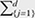wjxj = wTx, where w = (w1,...,wd) is a weight vector estimated from previously sold houses. By inspecting the estimated weights, we can infer what features influence house price the most. In addition, by using the aforementioned model equation, we can predict the price of new houses, given their features.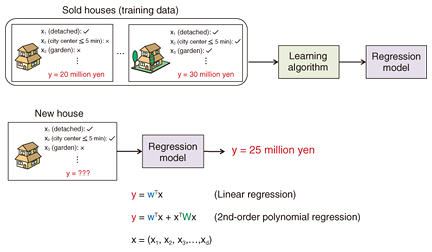Fig. 1. Application of regression analysis to house price prediction.

However, while linear regression is very simple, it has some limitations. For example, while the price of both detached and terraced houses decreases with distance from the city center, we expect the price of terraced houses to decrease faster than that of detached houses. In this case, since linear regression estimates a weight for the distance from city center independently of whether a house is detached or terraced, it cannot achieve high predictive accuracy. To solve this problem, it is necessary to estimate different weights for the distance to the city center, depending on whether a house is detached or terraced. In other words, it is necessary to introduce feature combinations in the model equation. This is called second-order polynomial regression.

Second-order polynomial regression can estimate models that fit the data better than linear regression. However, because the number of feature combinations is quadratic in the number of features, the number of feature combinations can quickly explode. For example, in genomic selection, which is the task of predicting grain yield from the DNA (deoxyribonucleic acid) of cereal plants, the number of genes is very large, and therefore, using feature combinations in the model equation can become impractical. Factorization machines (FM)  are a recently proposed method that can deal with a large number of feature combinations. Unfortunately, with FM, the quality of the estimated model strongly depends on the parameter initialization. To address this issue, we at NTT Communication Science Laboratories developed convex factorization machines (CFM), a new technology that can both cope with a large number of feature combinations and guarantees a globally optimal model regardless of the initialization .

## 2. CFM

In second-order polynomial regression, the relationship between house features and house price is modeled by the equation y = wTx + xTWx, where again, w is a weight vector, and W is a matrix whose elements correspond to the weights of feature combinations. When the number of features d is large, estimating W can quickly become impractical because W is a d x d matrix. To address this issue, both CFM and the original FM reduce the number of parameters to be estimated by assuming that W is a low-rank matrix. With the original FM, W is replaced by PPT, where P is a d x k matrix (k << d) and k is a user-defined rank hyper-parameter. The original FM then use training data to estimate P instead of W (Fig. 2). However, because the estimation of P involves a non-convex optimization problem, the quality of the obtained parameters greatly depends on the initialization. In practice, it is therefore necessary to try different initializations in order to obtain good results.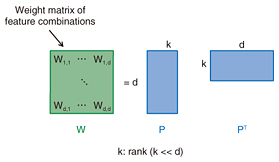Fig. 2. Matrix decomposition obtained by the original FM.

In contrast, our proposed technology, CFM, is guaranteed to obtain globally optimal model parameters regardless of the initialization. We developed an efficient algorithm to learn W in eigendecomposition form. We can use our algorithm to estimate the k eigenvalue-eigenvector pairs of W (Fig. 3). In addition, our algorithm automatically determines the rank k of W from data.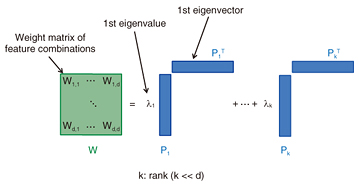Fig. 3. Eigendecomposition obtained by CFM.

In Table 1, we empirically compare ordinary second-order polynomial regression (i.e., without a low-rank constraint), FM, and CFM on genomic selection (the task of predicting grain yield from the DNA of cereal plants). The values in the table indicate the Pearson correlation between the true grain yield and the grain yield predicted by the three methods (higher is better) on test data. Results for FM were obtained by trying several possible initializations. These results show that CFM can achieve higher predictive accuracy than FM. In addition, the CFM results are also better than those for ordinary second-order polynomial regression. In machine learning, it is generally known that a model can overfit the data if the number of parameters is too large. We believe that CFM can mitigate this issue thanks to the reduced number of parameters to be estimated.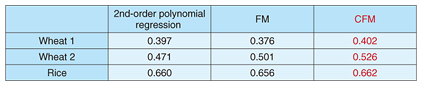Table 1. Example of application of CFM to genomic selection.

An important property of the low-rank constraint used in FM and CFM is that it enables the weights of feature combinations that were not observed in the training set to be estimated. This property is particularly useful in implementing recommender systems, a domain where FM have been particularly popular in recent years.

## 3. Higher-order extensions

We presented CFM, a new technology capable of efficiently leveraging second-order feature combinations. To further improve predictive accuracy, it is sometimes useful to consider third-order or higher-order feature combinations. We recently proposed new efficient algorithms for this purpose [3, 4].

## References

 S. Rendle, “Factorization Machines,” Proc. of ICDM 2010 (the 10th IEEE International Conference on Data Mining), pp. 995–1000, Sydney, Australia, Dec. 2010. M. Blondel, A. Fujino, and N. Ueda, “Convex Factorization Machines,” Proc. of ECML PKDD (European Conference on Machine Learning and Principles and Practice of Knowledge Discovery in Databases) 2015, Vol. 9285, pp. 19–35, Porto, Portugal, Sept. 2015. M. Blondel, M. Ishihata, A. Fujino, and N. Ueda, “Polynomial Networks and Factorization Machines: New Insights and Efficient Training Algorithms,” Proc. of ICML 2016 (the 33rd International Conference on Machine Learning), pp. 850–858, New York, USA, June 2016. M. Blondel, A. Fujino, N. Ueda, and M. Ishihata, “Higher-order Factorization Machines,” Proc. of NIPS 2016 (the 30th Annual Conference on Neural Information Processing Systems), to appear, Barcelona, Spain, Dec. 2016.Mathieu Blondel Research Scientist, Ueda Research Group, NTT Communication Science Laboratories. He received an engineering diploma from Telecom Lille, France, in 2008 and a Ph.D. in engineering from Kobe University, Hyogo, in 2013. He joined NTT Communication Science Laboratories in 2013. His current research interests include machine learning, mathematical optimization, the design of efficient machine learning software, and the application of these areas to real-world applications. Akinori Fujino Senior Research Scientist, Learning and Intelligent Systems Research Group, NTT Communication Science Laboratories. He received a B.E. and M.E. in precision engineering from Kyoto University in 1995 and 1997, and a Ph.D. in informatics from Kyoto University in 2009. He joined NTT in 1997. His current research interests include machine learning and knowledge discovery from complex data. Naonori Ueda Head of Ueda Research Laboratory, NTT Communication Science Laboratories and NTT Fellow. He received his B.S., M.S., and Ph.D. in communication engineering from Osaka University in 1982, 1984, and 1992. He joined Yokosuka Electrical Communication Laboratories of Nippon Telegraph and Telephone Public Corporation (now NTT) in 1984. In 1994, he moved to NTT Communication Science Laboratories in Kyoto, where he has been researching statistical machine learning, Bayesian statistics, and their applications to web data mining and big data analysis. From 1993 to 1994, he was a visiting scholar at Purdue University, Indiana, USA. He is a guest professor at the National Institute of Informatics and a visiting professor at Kyoto University. He is a Fellow of the Institute of Electronics, Information and Communication Engineers and a member of the Information Processing Society of Japan and the Institute of Electrical and Electronics Engineers. In July 2013, he became Director of Machine Learning Data Science Center, after serving as Director of NTT Communication Science Laboratories for three years. Since April, 2016, he has been head of Ueda Research Laboratory, NTT Communication Science Laboratories, and an NTT Fellow.

↑ TOP